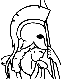#Network Optimization: Continuous and Discrete Models Table of Contents:

1. Introduction

1. Graphs and Flows
1. Paths and Cycles
2. Flow and Divergence
3. Path Flows and Conformal Decomposition
2. Network Flow Models -- Examples
1. The Minimum Cost Flow Problem
2. Network Flow Problems with Convex Cost
3. Multicommodity Flow Problems
4. Discrete Network Optimization Problems
3. Network Flow Algorithms -- An Overview
1. Primal Cost Improvement
2. Dual Cost Improvement
3. Auction
4. Good, Bad, and Polynomial Algorithms
4. Notes and Sources

2. Shortest Path Problems

1. Problem Formulation and Applications
2. A Generic Shortest Path Algorithm
3. Label Setting (Dijkstra) Methods
1. Performance of Label Setting Methods
2. The Binary Heap Method
3. Dial's Algorithm
4. Label Correcting Methods
1. The Bellman-Ford Method
2. The D'Esopo-Pape Algorithm
3. The SLF and LLL Algorithms
4. The Threshold Algorithm
5. Comparison of Label Setting and Label Correcting
5. Single Origin/Single Destination Methods
1. Label Setting
2. Label Correcting
6. Auction Algorithms
7. Multiple Origin/Multiple Destination Methods
8. Notes and Sources

3. The Max-Flow Problem

1. The Max-Flow and Min-Cut Problems
1. Cuts in a Graph
2. The Max-Flow/Min-Cut Theorem
3. The Maximal and Minimal Saturated Cuts
4. Decomposition of Infeasible Network Flow Problems
2. The Ford-Fulkerson Algorithm
3. Price-Based Augmenting Path Algorithms
1. A Price-Based Path Construction Algorithm
2. A Price-Based Max-Flow Algorithm
4. Notes and Sources

4. The Min-Cost Flow Problem

1. Transformations and Equivalences
1. Setting the Lower Flow Bounds to Zeros
2. Eliminating the Upper Flow Bounds
3. Reduction to a Circulation Format
4. Reduction to an Assignment Problem
2. Duality
1. Interpretation of CS and the Dual Problem
2. Duality and CS for Nonnegativity Constraints
3. Notes and Sources

5. Simplex Methods for Min-Cost Flow

1. Main Ideas in Simplex Methods
1. Using Prices to Obtain the In-Arc
2. Obtaining the Out-Arc
3. Dealing with Degeneracy
2. The Basic Simplex Algorithm
1. Termination Properties of the Simplex Method
2. Initialization of the Simplex Method
3. Extension to Problems with Upper and Lower Bounds
4. Implementation Issues
5. Notes and Sources

6. Dual Ascent Methods for Min-Cost Flow

1. Dual Ascent
2. The Primal-Dual (Sequential Shortest Path) Method
3. The Relaxation Method
4. Sensitivity Analysis
5. Implementation Issues
6. Notes and Sources

7. Auction Algorithms for Min-Cost Flow

1. The Auction Algorithm for the Assignment Problem
1. The Main Auction Algorithm
2. The Approximate Coordinate Descent Interpretation
3. Variants of the Auction Algorithm
4. Computational Complexity -- \$\e\$-Scaling
5. Dealing with Infeasibility
2. Extensions of the Auction Algorithm
1. Reverse Auction
2. Auction Algorithms for Asymmetric Assignment
3. Auction Algorithms with Similar Persons
3. The Preflow-Push Algorithm for Max-Flow
1. Analysis and Complexity
2. Implementation Issues
3. Relation to the Auction Algorithm
4. The \$\e\$-Relaxation Method
1. Computational Complexity -- \$\e\$-Scaling
2. Implementation Issues
5. The Auction/Sequential Shortest Path Algorithm
6. Notes and Sources

8. Nonlinear Network Optimization

1. Separable Convex Problems Problems
2. Multicommodity Flows
3. Side Constraints
4. Integer Constraints
5. Networks with Gains
6. Optimality Conditions
7. Duality
8. Algorithms and Approximations
1. Feasible Direction Methods
2. Piecewise Linear Approximation
3. Interior Point Methods
4. Penalty and Augmented Lagrangian Methods
5. Proximal Minimization
6. Smoothing
7. Transformations
9. Notes and Sources

9. Convex Separable Network Problems

1. Convex Functions of a Single Variable
2. Optimality Conditions
3. Duality
4. Dual Function Differentiability
5. Algorithms for Differentiable Dual Problems
6. Auction Algorithms
1. The \$\e\$-Relaxation Method
2. The Auction/Sequential Shortest Path Algorithm
7. Monotropic Programming
8. Notes and Sources

10. Network Problems with Integer Constraints

1. Formulation of Integer-Constrained Problems
2. Branch-and-Bound
3. Lagrangian Relaxation
2. Cutting Planes
3. Decomposition Methods for Multicommodity Flows
4. Local Search Methods
1. Tabu Search
2. Genetic Algorithms
3. Simulated Annealing
5. Rollout Algorithms
6. Notes and Sources

11. References

12. Index